Figures for "Harmonograms"

Cameron Browne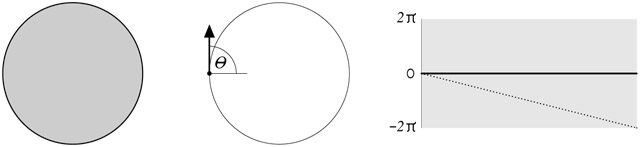Figure 1. A circle, a starting point on its boundary, and its angular profile.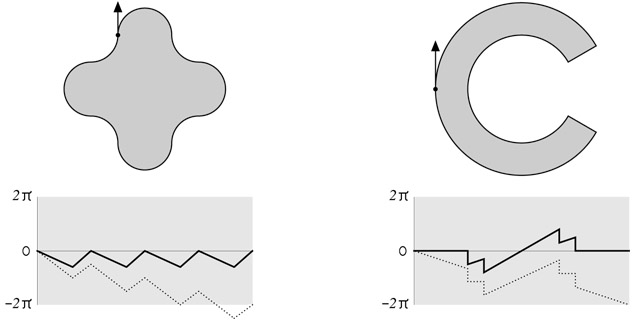Figure 2. Two closed planar shapes and their angular profiles.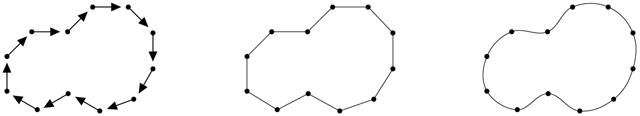Figure 3. Sampling at regular intervals, polygonal approximation, and smooth spline curve.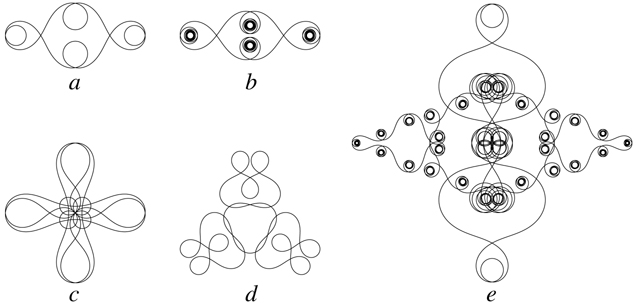Figure 4. Reconstructible harmonograms: "e"2,1, "!"2,1, "cb"4,1, " ab"3,1, and "pen and ink"2,1.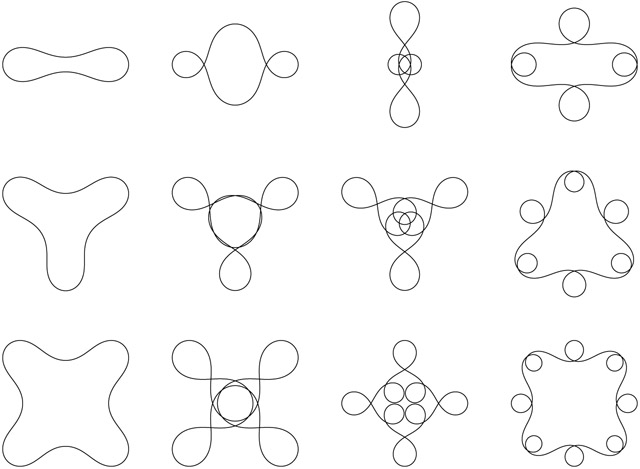Figure 5. Effect of m and s. Top row: "a"2,1..4. Middle row: "a"3,1..4. Bottom row: "a"4,1..4.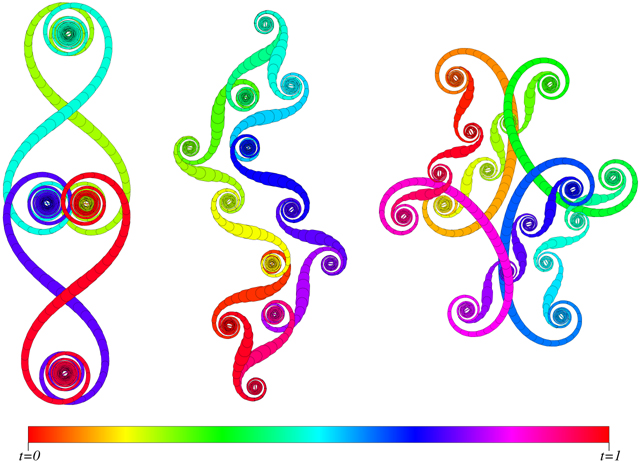Figure 6. The curve "Z"2,166 at >= 2,500 samples, and undersampled at 1,250 and 1,000 samples.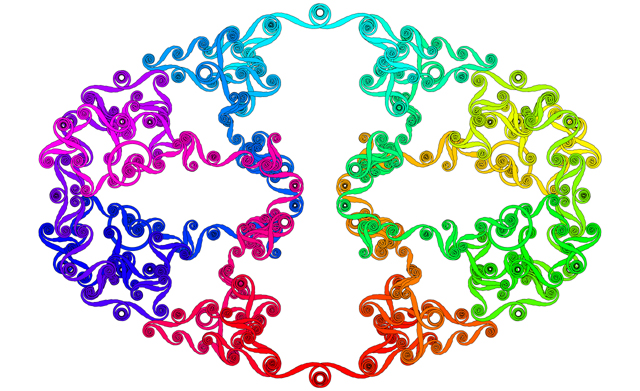Figure 7. "What's the buzz?"2,17 at 11,014 samples.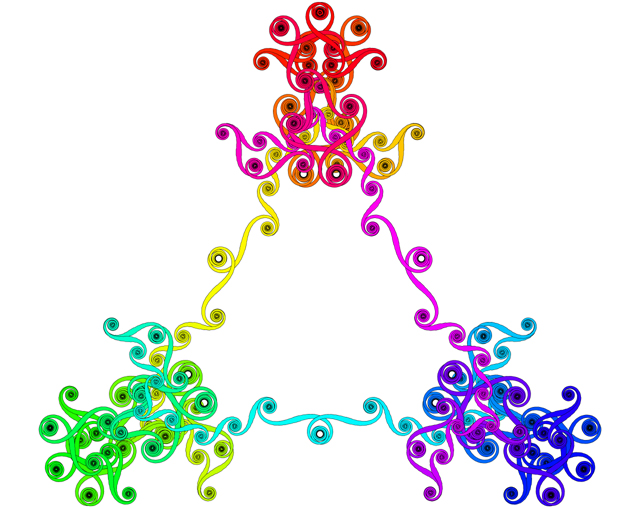Figure 8. A picture of " you"3,117 at 12,003 samples.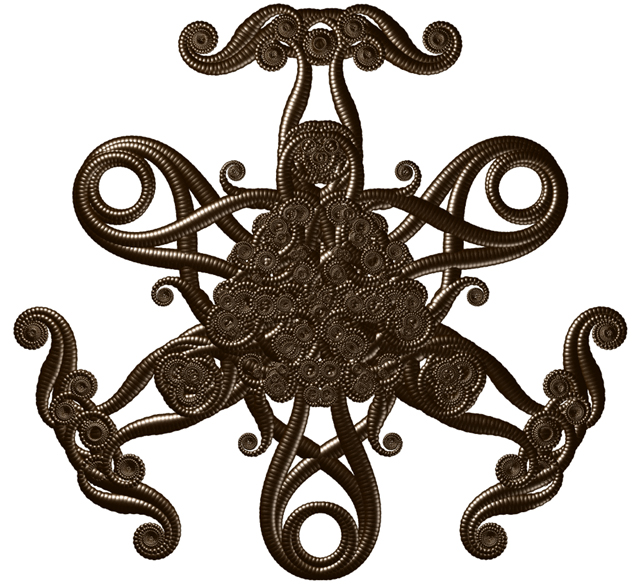Figure 9. The darker side of " you"3,117 at 14,004 samples (POV-Ray polysphere).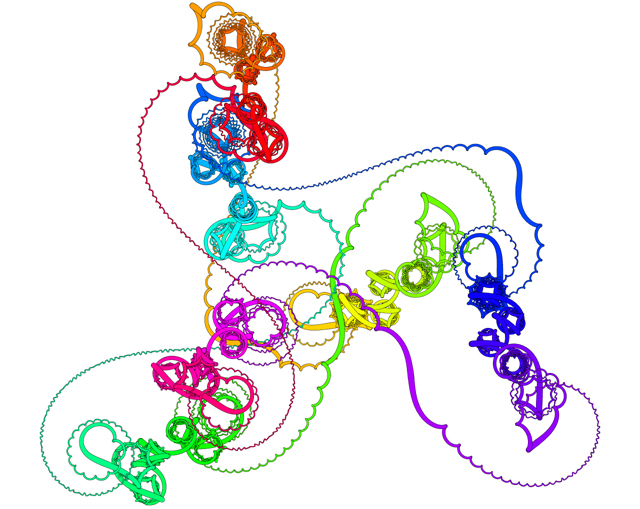Figure 10. A clockwork bicycle: "a"3,141 + "a"7,2102 at 10,000 samples.